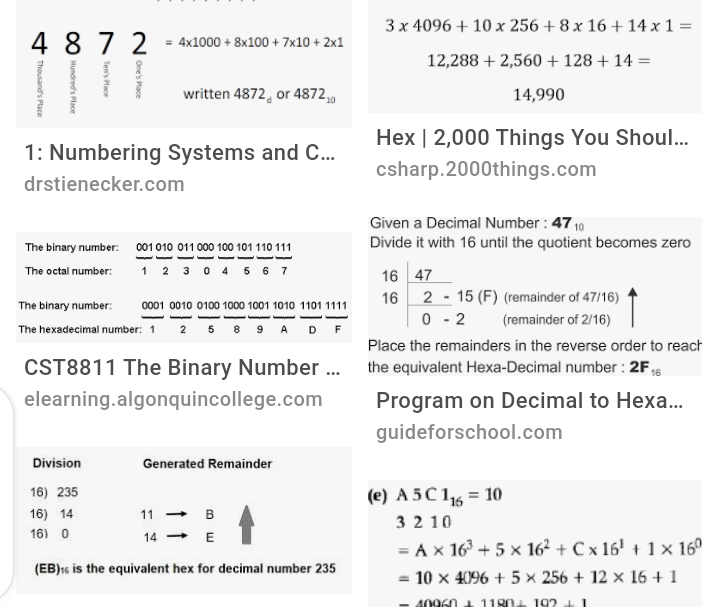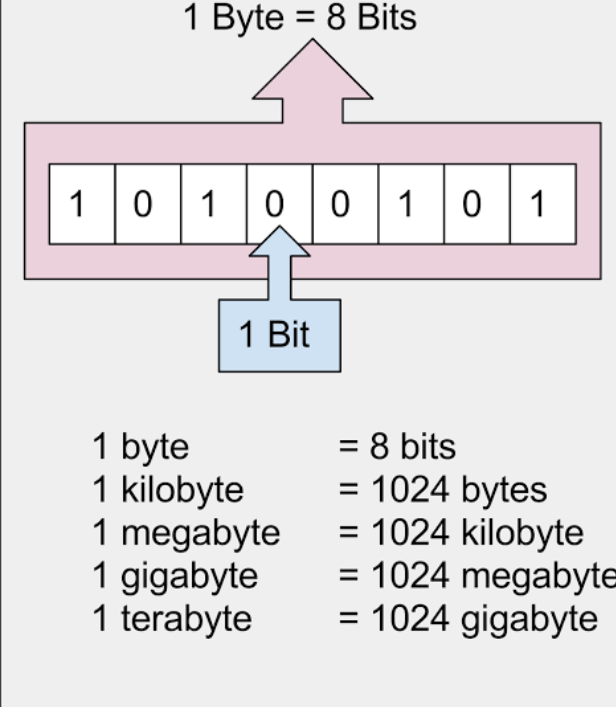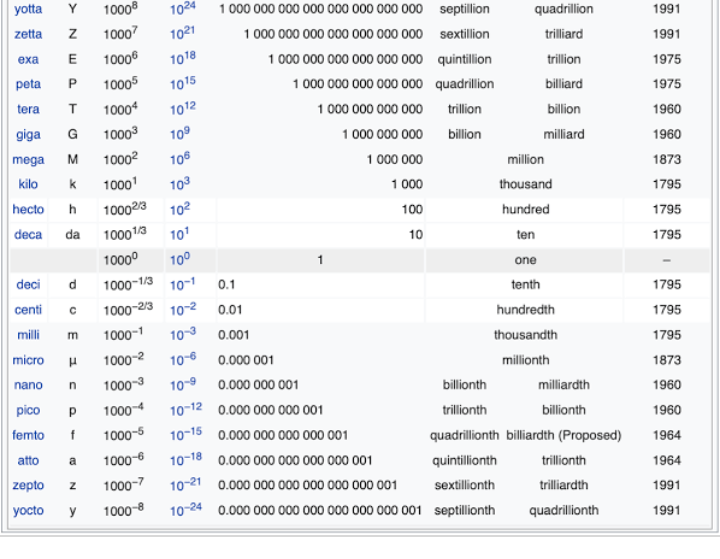Sign inMathematics: Easy way to convert numbers in base two to octal and hexadecimal forms

Mathematics is an important tool in Computer Science. In fact, computation exploits Mathematical tools to solve problems most of the times.

Based on this, it has become a good suggestion that a computer scientist have skill in Mathematics.

Nevertheless, this post is intended to address some important skill in Computer Science: Number System.NUMBER SYSTEM

Computer solves problems in its language. The language of computer is known as Machine Language. Machine Language is representation of software items in combination of zeros and ones(0,1) as bytes, with a byte equals to 8 bits.

For instance, 1 is represented as 00000001 in computer memory in 8 bit system which is equivalent to a byte of computer memory. Computer memory being arrays: matrix-like structure for element representation and storage.

Decimal number 5 is represented as 00000101 in 8 bit system. This contiguous combination of 0s and 1s are either in base 2 or 8 or 16.Conversion from base 2 to Octal (base 8)

Example:

Given a base two(binary) number 10001; to convert it to base eight, we follow this steps:

1. Start from right side, divide the digits in pairs of 3 digits.

2. Convert each pair to decimal number

3. Combine the resulting numbers, and that is the equivalent in base eight.

For our example:

10001 == 10 001

10 == 010 (add one 0 to make it 3 digits)

Hence, 10001 == 010 001

To base 10,

010 = 2 and 001 = 1

Therefore, 10001 in base 2 is equal to 21 in base 8.Conversion of binary numbers to base 16(Hexadecimal numbers)

Hexadecimal numbers have digits in 1,2,3,4,5,6,7,8,9,A,B,C,D,E,F.

A stands for 10;

B for 11;

C for 12;

D for 13;

E for 14;

F for 15

For binary numbers to be converted to hexadecimal numbers, steps below are needed.

1. Divide the digits into pairs of four digits starting from the right side.

2. Convert each pair to base 10.

3. Combine the resulting decimal numbers, and that is the hexadecimal equivalent.

Example: Convert 100111100 in base 2 to base 16(hexadecimal.

Solution:

Step 1:

100111100 : 1 0011 1100

: 0001 0011 1100

To decimal :. 1 3. 12

To hexadecimal: 13C, recall that C is equivalent to 12.

That was easy ways to convert binary numbers to octal and hexadecimal.

Watch out for how to convert vice versa in subsequent posts I would be released on Computer tech.

Thanks.

Content created and supplied by: WakeUpNow (via Opera News )

Computer Science Machine Language